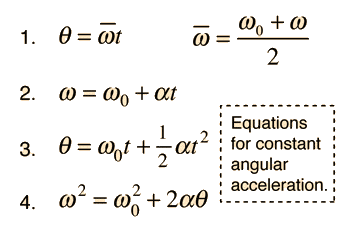# Angular Acceleration of a Moving Wheel

• underoathP

#### underoathP

I am having a bit of trouble with the following problem.

A cyclist starts from rest and pedals so that the wheels make 8.5 revolutions in the first 4.6 s. What is the angular acceleration of the wheels (assumed constant)?

I multiplied 8.5 by 2pi and then divided by 4.6 sec. I get an answer of 11.6 rad/sec, but they want an answer in rad/sec^2. Am I doing this right?

I am having a bit of trouble with the following problem.

A cyclist starts from rest and pedals so that the wheels make 8.5 revolutions in the first 4.6 s. What is the angular acceleration of the wheels (assumed constant)?

I multiplied 8.5 by 2pi and then divided by 4.6 sec. I get an answer of 11.6 rad/sec, but they want an answer in rad/sec^2. Am I doing this right?
I see you've found the Homework Forums,.

Not quite. What is the definition of angular acceleration?

Last edited:
Angular Acceleration

The definition of angular acceleration is angular velocity over time. So, to get angular velocity from 8.5 rev/4.6 sec you have to find the angular velocity first. So, the definition of angular velocity (omega) is angular displacement over time, and the angular displacement is in radians. That is why I multiplied 8.5 by 2pi. Do I have to divide by 4.6 again?

I looked at that website and this is what makes sense to me:

8.5 rev * 2pi = 53.41 radians

2.5 is the wrong answer when I entered it. I am out of ideas.

I looked at that website and this is what makes sense to me:

8.5 rev * 2pi = 53.41 radians

2.5 is the wrong answer when I entered it. I am out of ideas.